# Towards uncertain network optimization

## Abstract

Uncertain network optimization is the study of network optimization with uncertain data which we often meet in decision making under the presence of uncertainties. The main purpose of this manuscript is to present a state-of-the-art review on the recent advances in uncertain network optimization and to show the general uncertain network optimization models based on an uncertainty theory. Some classical network optimization topics in an uncertain environment are revisited, and some challenging topics in future research are addressed in the field of uncertain network optimization. This paper divides uncertain network optimization into two main directions. One line of research deals with network optimization problems with an uncertain topology structure, and another line of research concerns the handling of network optimization with uncertain weights.

## Introduction

In real life, we are faced with so many networks such as road network, telecommunication network, television network, computer network, logistics network, social network, and so on. In practice, a lot of network optimization problems [1,2] are issued from a wide variety of the networks. Generally speaking, network optimization is to study the problems on how to design, manage, control, and optimize the network efficiently. As we can see, there are many variations of typical network optimization problems such as shortest path problem , minimum spanning tree , transportation problem , assignment problem , maximum flow problem , and so forth. These attractive problems have motivated many researchers to model the network optimization problems mathematically.

In the abovementioned research, the network optimization problems were usually investigated in a deterministic environment, in which the capacities of the arcs, the costs of the transportation, etc., are treated as positive crisp values. However, some indeterminacy factors might occur in the problems. In fact, due to the lack of adequate sample data, or the detail sample data are not easy to get because of economic reasons or technical difficulties, the capacities of the arcs and the costs of the transportation are not sharply known in advance. As a result, the capacity and the cost are described by some empirical data such as ‘about 2 tons’ and ‘approximately 3 dollars,’ respectively. In this situation, it is not suitable to employ the classical models and algorithms to study the network optimization problems directly.

Probabilistic approaches are borrowed in the analysis of network optimization problems. Moreover, some researchers employed probability theory to investigate the stochastic network optimization problems, the stochastic versions of the network optimization problems. Williams  proposed a stochastic transportation model, in which the demands were supposed to be random variables. Donath  investigated random assignment problems. Hall  concerned a problem of finding the least expected travel time path between two vertices in a network with travel times that are both random and time-dependent. Nawathe and Rao  provided a general approach for the maximum flow problem in probabilistic communication network. Mulvey and Vladimirou  described the stochastic network optimization models for investment planning. Focusing on communication and queueing systems, Neely  presented a modern theory of analysis, control, and optimization for stochastic networks. In , Peer and Sharma defined a probabilistic shortest path problem and formulated it as a linear programming problem. Ji et al.  proposed a new simulation-based multi-objective genetic algorithm approach to find a portfolio of reliable paths in stochastic networks.

In addition, some optimization problems were founded in the literature on fuzzy network, such as Chanas and Kolodziejczyk , Hanebeck and Schmidt , and Wu .

Essentially, the indeterminacy phenomena can be divided into two distinct types according to the present mathematical systems to deal with them. One is stochastic phenomena, and another is uncertain phenomena. When the sample size is too small or even no sample to estimate a probability distribution, we have to invite some domain experts to evaluate their belief degree that each event will occur. A lot of surveys showed that human beings usually estimate a much wider range of values than the object actually takes . Probability theory is inappropriate in this case because it may lead to counterintuitive results . In order to distinguish from randomness, this phenomenon was named uncertainty . In order to deal with the uncertain phenomena, Liu  founded an uncertainty theory, which has become a branch of axiomatic mathematics for modeling human uncertainty.

In theoretical aspect, uncertain set (Liu [22,23]), uncertain calculus (Liu , Yao ), and uncertain differential equation (Liu , Chen and Liu , Yao ) have been established. From a practical aspect, uncertain finance (Liu , Peng , Peng and Yao ), uncertain inference (Liu , Gao et al. ), uncertain graph (Gao and Gao , Zhang and Peng [34,35]), etc., have also been developed. As a mathematical programming involving uncertain variables, uncertain programming was introduced by Liu  in 2009. After that, many scholars employed uncertain programming to some optimization problems in the real world. Yan  introduced portfolio selection in an uncertain environment and proposed two uncertain models. Rong  investigated economic order quantity for inventory based on uncertain programming. Gao  studied shortest path problem with uncertain lengths. Zhang and Chen  introduced uncertain programming to project scheduling problem. Furthermore, uncertain programming have also been used in supply chain design (Ding ), facility location problem (Gao ), parallel machine scheduling (Zhang and Meng ), and inverse minimum spanning tree problem (Zhang et al. [44,45]).

As an interdisciplinary subject of combinatorial optimization and uncertainty theory, we are interested in considering uncertain network optimization problem. There are two types of uncertain network optimization problems. One is network optimization with an uncertain topology structure. In order to deal with these kinds of uncertain factors, an uncertain graph was proposed by Gao and Gao . After that, Euler tour , cycle index , Hamilton cycle , matching , connectivity of two vertices , and diameter  for an uncertain graph were considered by scholars. Another research field concerns network optimization problem with uncertain weights, including Chinese postman problem , network flow problem [51,52], optimal assignment problem [53,54], minimum spanning tree problem [55,56], and shortest path problem [39,57].

Instead of surveying all the researches on uncertain network optimization problems so far, this paper intends to provide an overview of an uncertain graph with uncertain edges and uncertain programming models which deal with uncertain network optimization problems based on an uncertain measure. The authors hope to provide the readers with some ideas to deal with uncertain factors in a graph and several basic types of uncertain network optimization models.

The rest of this paper is organized as follows. In the ‘Preliminary’ section, some necessary preliminary concepts and results extracted from uncertainty theory will be presented. After that, the classical network optimization problem and stochastic network optimization problems are introduced. Following that, network optimization with an uncertain topology structure is presented. In addition, we introduce network optimization with uncertain weights, including three kinds of uncertain programming models. What is more, we study some typical uncertain network optimization problems. Then a hybrid intelligent algorithm for solving the optimization models in general cases is introduced. After some topics on further research, some remarks are given in the last section.

## Preliminary

As an efficient tool of modeling the behavior of uncertain phenomena, the uncertainty theory is employed to deal with uncertain network optimization problems. Uncertainty theory, proposed by Liu , is a branch of mathematics based on normality, duality, subadditivity, and product axioms. In this section, we are particularly interested in some related preliminaries from the uncertainty theory.

The first fundamental concept in the uncertainty theory is the uncertain measure that is interpreted as the personal belief degree (not frequency) of an uncertain event that may occur.

Let Γ be a nonempty set, and L a σ-algebra over Γ. For any ΛL, Liu  presented an axiomatic uncertain measure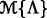to express the chance that uncertain event Λ occurs. The set function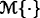satisfies the following three axioms:

(i) (Normality)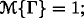; (i i) (Duality)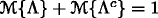for any ΛL; (i i i) (Subadditivity) For every countable sequence of events {Λ i }, we have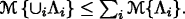For the sake of convenience, the triplet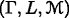is called an uncertainty space. Liu  defined a product of the uncertain measure by way of the fourth axiom of uncertainty theory, which makes major differences in operations between uncertainty theory and probability theory. Let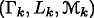be uncertainty spaces for k=1,2, Write

$$\Gamma=\Gamma_{1}\times\Gamma_{2}\times\cdots,\quad L=L_{1}\times L_{2}\times\cdots$$

Then the product uncertain measure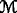on the product σ-algebra L is defined by the following axiom:

(i v) (Product Axiom)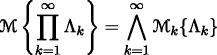where Λ k are arbitrarily chosen events from L k for k=1,2,, respectively.

An uncertain variable is defined by Liu  as a measurable function from an uncertainty space to the set of real numbers. An uncertain variable ξ can be characterized by its uncertainty distribution Φ:→[0,1], which is defined by Liu  as follows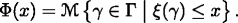Let ξ be an uncertain variable with uncertainty distribution Φ. Then the inverse function Φ −1 is naturally called the inverse uncertainty distribution of ξ.

The expected value of uncertain variable ξ is mathematically defined by Liu  as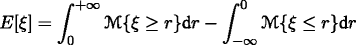provided that at least one of the two integrals is finite.

### Theorem1.

(Liu ) Let ξ be an uncertain variable with uncertainty distribution Φ. If its expected value exists, then

$$E[\xi]={\int_{0}^{1}}\Phi^{-1}(\alpha)\textit{d}\alpha$$

where Φ −1 is the inverse uncertainty distribution of uncertain variable ξ.

Liu  introduced the independence concept of uncertain variables in the following way. The uncertain variables ξ 1,ξ 2,,ξ m are independent if and only if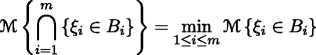for any Borel sets B 1,B 2,,B m of .

A real-valued function of multiple variables f(x 1,x 2,,x n ) is said to be strictly increasing if

$$f(x_{1},x_{2},\cdots,x_{n})\leq f(y_{1},y_{2},\cdots,y_{n})$$

whenever x i y i for i=1,2,,n and

$$f(x_{1},x_{2},\cdots,x_{n})<f(y_{1},y_{2},\cdots,y_{n})$$

whenever x i <y i for i=1,2,,n.

Liu  provided the following useful theorem to determine the distribution function of the strictly increasing function of uncertain variables. Taking advantage of this theorem, we can transform an indeterminacy model into a deterministic one.

### Theorem2.

(Liu ) Let ξ 1,ξ 2,,ξ n be independent uncertain variables with uncertainty distributions Φ 1, Φ 2, , Φ n , respectively. If f is a strictly increasing function, then ξ=f(ξ 1,ξ 2,,ξ n ) is an uncertain variable with inverse uncertainty distribution

$$\Phi^{-1}(\alpha)=f(\Phi_{1}^{-1}(\alpha),\Phi_{2}^{-1}(\alpha),\cdots,\Phi_{n}^{-1}(\alpha)).$$

### Example1.

Let ξ 1 and ξ 2 be independent uncertain variables with uncertainty distributions Φ 1 and Φ 2, respectively. Since f(x 1,x 2)=x 1+ exp(x 2)is a strictly increasing function, ξ 1+ exp(ξ 2) is an uncertain variable with inverse uncertainty distribution

$$\Psi^{-1}(\alpha)=\Phi^{-1}_{1}(\alpha)+\exp(\Phi^{-1}_{2}(\alpha)).$$

### Example2.

Let ξ 1,ξ 2 and ξ 3 be independent and nonnegative uncertain variables with uncertainty distributions Φ 1,Φ 2 and Φ 3, respectively. Since $$f(x_{1},x_{2},x_{3})={x_{1}^{2}}+x_{2}\times x_{3}$$ is a strictly increasing function, $${\xi _{1}^{2}}+\xi _{2}\times \xi _{3}$$ is an uncertain variable with inverse uncertainty distribution

$$\Psi^{-1}(\alpha)=(\Phi_{1}^{-1}(\alpha))^{2}+\Phi_{2}^{-1}(\alpha)\times \Phi_{3}^{-1}(\alpha).$$

## Network optimization

### Classical network optimization problem

Classical network optimization problems, including shortest path problem, maximum flow problem, transportation problem, matching problem, etc., have been researched for a long time and resulted in many gratifying achievements . In a classical network optimization problem, the topology structure of the network is deterministic and the parameters in the network are crisp. With ignoring so complicated factors, the classical network optimization problems have permeated every aspect of our lives, and the models of these optimization problems have been widely used in the applications in the real world.

As we know, a network optimization problem is usually described as a special type of linear programming model. For simplicity, we use x=(x 1,x 2,,x n ) to represent the decision vector. Assume that f(x) is the objective function of the network optimization, and the constrained conditions from the network can be expressed as g j (x)≥0 for j=1,2,,m. Then the classical network optimization model can be formulated as

$$\begin{array}{c} \left\{\begin{array}{ll} \min \quad \displaystyle f(\textbf{x}) \\ \text{subject to}:\\ \quad\quad\quad \displaystyle g_{j}(\textbf{x})\ge 0, &\quad j=1,2,\cdots,m \\ \quad\quad\quad \textbf{x}\in D \end{array}\right. \end{array}$$

where D emphatically denotes the restricted region for the sake of clarity.

### Stochastic network optimization problem

Many real-life networks behave not deterministic but stochastic. Examples can be found in areas like communication systems, production, maintenance, and logistics systems. Construction and analysis of stochastic network optimization models rely on techniques from fundamental probability theory and mathematical statistics.

In stochastic network optimization, it is assumed that x is a decision vector, ξ is a random vector related to random variables, and f(x; ξ) is the objective function to be optimized stochastically. Suppose that we have a set of random constraints g j (x; ξ)≥0, j=1,2,,m.

Generally, a network optimization problem with random variables can be described by a stochastic programming model. In order to obtain a decision with minimum expected objective value subject to a set of chance constraints, the stochastic network optimization problem can be expressed as

$$\begin{array}{c} \left\{\begin{array}{ll} \min \quad \displaystyle E[f(\textbf{x};\text{\boldmath{$$\xi$$}})] \\ \text{subject to}:\\ \quad\quad\quad \displaystyle \text{Pr}\{g_{j}(\textbf{x};\text{\boldmath{$$\xi$$}})\ge 0\}\geq \alpha_{j}, &\quad j=1,2,\cdots,m \\ \quad\quad\quad \textbf{x}\in D \end{array}\right. \end{array}$$

where α j ,j=1,2,,m are the predetermined confidence levels, and D is the restricted region.

As mentioned before, there are two kinds of indeterminacy. Probability theory was developed by Kolmogorov  for modeling frequencies, and uncertainty theory was founded by Liu  for modeling belief degrees. This paper aims at providing the general methodology of modeling uncertain network optimization problems rather than surveying the up-to-date advances in uncertain network optimization, in which the uncertain factors are dealt with by the uncertain measure. In this paper, we provide the uncertain network optimization problems in two main directions. One line of research concerns the handling of network with the uncertain topology structure, which is called an uncertain graph. Another line of research deals with network optimization with uncertain weights, such as an uncertain Chinese postman problem , uncertain assignment problem [53,54], and uncertain transportation problem [61-63].

## Network optimization with an uncertain topology structure

In a classic graph theory, the vertices and edges are deterministic, either existing or not. However, with different scenarios from stochastic ones, very often we are lack of observed data due to economic reasons or technical difficulties. As we stated before, when there do not exist enough information for us to judge whether two nodes are joined by an edge, we usually use the belief degree to describe it. Since human beings usually overweigh unlikely events , the belief degree may have much larger variance than the real frequency; these belief degree data are no more suitable to be dealt with by probability theory. A counterexample was presented by Zhang and Peng .

Based on the analysis mentioned above, Gao and Gao  defined the uncertain graph, in which all edges are independent and exist with some belief degrees in an uncertain measure.

### Definition1.

(Gao and Gao ) A simple finite graph of order n is said to be uncertain if its adjacency matrix is

$$A=\left(\begin{array}{cccc} 0 & \alpha_{12} & \cdots & \alpha_{1n} \\ \alpha_{21} & 0 & \cdots & \alpha_{2n} \\ \vdots & \vdots & \ddots & \vdots \\ \alpha_{n1} & \alpha_{n2} & \cdots & 0 \end{array} \right)$$

where α ij represents the truth values in the uncertain measure that the edges between vertices v i and v j exist, i,j=1,2,,n, respectively.

After that, Gao and Gao  discussed the connectivity of the uncertain graph. Briefly, a graph G is connected if there is a uv path whenever two vertices u,v of G. For the uncertain graph, in order to show how likely the uncertain graph is connected, Gao and Gao  defined connectedness index as below.

### Definition2.

(Gao and Gao ) A connectedness index of an uncertain graph is the uncertain measure that the uncertain graph is connected.

In addition, a method for calculating connectedness index was given.

### Theorem3.

(Gao and Gao ) Let G be an uncertain graph of order n and its uncertain adjacency matrix is

$$A=\left(\begin{array}{cccc} 0 & \alpha_{12} & \cdots & \alpha_{1n} \\ \alpha_{21} & 0 & \cdots & \alpha_{2n} \\ \vdots & \vdots & \ddots & \vdots \\ \alpha_{n1} & \alpha_{n2} & \cdots & 0 \end{array} \right).$$

If all edges are independent, then the connectedness index of G is

$$\rho(G)=\left\{ \begin{array}{l} \,\displaystyle{\sup_{f(X)=1}}\displaystyle{\min_{1\leq i<j\leq n}\nu_{ij}(X)}, \:\:\quad\quad{\text{if} \displaystyle{\sup_{f(X)=1}}\displaystyle{\min_{1\leq i<j\leq n}\nu_{ij}(X)}<0.5}\\ \,1-\displaystyle{\sup_{f(X)=0}}\displaystyle{\min_{1\leq i<j\leq n}\nu_{ij}(X)},\:\:\;{\text{if} \displaystyle{\sup_{f(X)=1}}\displaystyle{\min_{1\leq i<j\leq n}\nu_{ij}(X)}\geq0.5} \end{array} \right.$$

where

$$X=\left(\begin{array}{cccc} x_{11} & x_{12} & \cdots & x_{1n} \\ x_{21} & x_{22} & \cdots & x_{2n} \\ \vdots & \vdots & \ddots & \vdots \\ x_{n1} & x_{n2} & \cdots & x_{nn} \end{array} \right),$$

x ij {0,1}, and ν ij are defined by

$$\nu_{ij}(X)=\left\{ \begin{array}{cl} \alpha_{ij},&{\text{if $$x_{ij}=1$$}}\\ 1-\alpha_{ij},&{\text{if $$x_{ij}=0$$}} \end{array} \right.$$

for i,j=1,2,,n, respectively,

$$f(X)=\left\{ \begin{array}{ll} 1,&\text{if each element of $$I+X+X^{2}+\cdots+X^{n-1}$$ is positive}\\ 0,&{\text{otherwise.}} \end{array} \right.$$

For more research of the uncertain graph, we may consult Gao , Gao et al. , Zhang and Peng [47,48], and so on. Nevertheless, there are many new and fundamental topics of the uncertain graph needed to be discussed.

## Network optimization with uncertain weights

Assume that the uncertain network is denoted by N=(V,A, ξ), where V is the node set, A is the edge set, and the uncertain vector ξ=(ξ 1,ξ 2,,ξ n ) stands for the uncertain values corresponding to the edge vector (e 1,e 2,,e n ). Without loss of generality, ξ i are considered independent uncertain variables with uncertainty distributions Φ i , i=1,2,,n, respectively.

For simplicity, we use x=(x 1,x 2,,x n ) to represent the decision vector, D to denote the restricted region. Assume the objective function is f(x; ξ). And the aim of the uncertain network optimization problem is to minimize f(x; ξ). The constrained conditions can be originally expressed as g j (x; ξ)≤0 for j=1,2,,m. In accordance with the practical problems, it is also assumed that g j (x; ξ) are strictly increasing with respect to ξ 1,ξ 2,,ξ n .

### Expected value model

In uncertain network optimization, it is difficult to optimize the uncertain objective value directly. Then an expected value model can be used to obtain a decision with an optimal expected objective value subject to the expected constraints.

Taking this point of view, we may minimize the expected value of the objective function, i.e.,

$$\min \quad E[f(\textbf{x};\text{\boldmath{$$\xi$$}})].$$

To make sense, the uncertain constraints g j (x; ξ)≤0 for j=1,2,,m should be expressed as:

$$E[g_{j}(\textbf{x};\text{\boldmath{$$\xi$$}})]\leq 0,\qquad j=1,2,\cdots,m.$$

Based on this modeling idea, the expected value model was constructed by Liu :

$$\begin{array}{c} \left\{\begin{array}{ll} \min \quad E[f(\textbf{x};\text{\boldmath{$$\xi$$}})] \\ \text{subject to}:\\ \quad\quad\quad E[g_{j}(\textbf{x};\text{\boldmath{$$\xi$$}})]\leq 0, &\quad j=1,2,\cdots,m\\ \quad\quad\quad \textbf{x}\in D. \end{array}\right. \end{array}$$
((1))

Taking advantage of properties of the operational law of the uncertain variable, the model (1) can be represented as

$$\begin{array}{c} \left\{\begin{array}{ll} \min \quad {\displaystyle\int_{0}^{1}}\Phi_{f}^{-1}(\textbf{x};\alpha)\mathrm{d}\alpha \\ \text{subject to}:\\ \quad\quad\quad {\displaystyle\int_{0}^{1}}\Psi_{g_{j}}^{-1}(\textbf{x};\alpha)\mathrm{d}\alpha\leq 0, &\quad j=1,2,\cdots,m\\ \quad\quad\quad \textbf{x}\in D \end{array}\right. \end{array}$$

where $$\Phi _{f}^{-1}$$ and $$\Psi _{g_{j}}^{-1}$$ are the inverse uncertainty distributions of f(x; ξ) and g j (x; ξ), respectively.

### Belief degree-constrained programming model

Belief degree-constrained programming is another method to deal with optimization problem in an uncertain environment. In order to make a decision so that the objective function f(x; ξ) is minimized in the sense of an uncertain measure, the α criteria can be taken as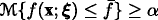where the critical value $$\bar {f}$$ will be minimized, and α is a predetermined confidence level.

In addition, it is naturally desired that the uncertain constraints g j (x;ξ)≤0 for j=1,2,,m hold with confidence levels α 1,α 2,,α m , respectively. Then the constrained conditions can be expressed by chance constraints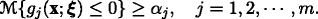Thus, Liu  presented the uncertain network optimization model as follows: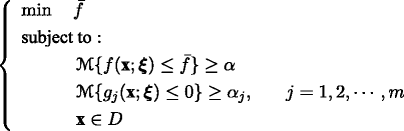((2))

where α and α j are the predetermined confidence levels.

Taking advantage of properties of the operational law of an uncertain variable, the model (2) can be equivalently represented as

$$\left\{\begin{array}{ll} \min \quad \Phi_{f}^{-1}(\textbf{x};\alpha) \\ \text{subject to}:\\ \quad\quad\quad \Psi_{g_{j}}^{-1}(\textbf{x};\alpha_{j})\leq 0, &\quad j=1,2,\cdots,m\\ \quad\quad\quad \textbf{x}\in D \end{array}\right.$$

where $$\Phi _{f}^{-1}$$ and $$\Psi _{g_{j}}^{-1}$$ are the inverse uncertainty distributions of f(x; ξ) and g j (x; ξ), respectively.

### Belief degree maximized-constrained programming model

Since there is no way to compare the uncertain objective functions directly, the decision maker may consider the problem from another point of view. The third criterion for the decision maker is a belief degree rule. The decision maker may firstly present a satisfying predetermined maximal value $$\bar {f}$$ and then maximize the belief degree that the objective function f(x; ξ) is no more than the given value. In the sense of belief degree, the constrains can be expressed by chance constraints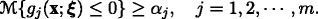Taking this modeling idea, Liu  constructed belief degree maximized-constrained programming model as follows: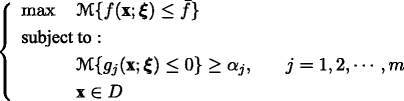((3))

where α j are the predetermined confidence levels.

Equivalently, it can be rewritten as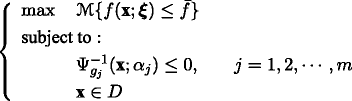where $$\Psi _{g_{j}}^{-1}$$ are the inverse uncertainty distributions of g j (x; ξ).

## Some uncertain network optimization problems

In this section, we focus on some typical uncertain network optimization problems to show the applications of the proposed models.

### Uncertain Chinese postman problem

Assume N=(V,A, ξ) is an uncertain network, where V={1,2,,n} is the set of nodes, A={(i,j)|i,jV,ij} is the set of edges, and ξ={ξ ij |(i,j)A,ij} is the set of the uncertain length of the edges. We also assume that ξ ij are independent uncertain variables with uncertainty distributions Φ ij , i,j=1,2,,n, respectively. The Chinese postman problem is to find a shortest route R such that the postman must cover each street in his area at least once, and then end up at the same place where he begin his route. Without loss of generality, we assume x ij is a decision variable on the edge (i,j). That is to say, x ij =1 means that there exists an edge from i to j in route R; Otherwise, x ij =0. In order to obtain a shortest route in the sense of expected value, Zhang and Peng  proposed the following expected value model,

$$\begin{array}{c} \left\{\begin{array}{ll} \min \quad E\left[\displaystyle{\sum_{(i,j)\in A}x_{ij}\xi_{ij}}\right] \\[0.3cm] \text{subject to}:\\ \quad\quad\quad \displaystyle{\sum_{j:(i,j)\in A}x_{ij}}-\displaystyle{\sum_{j:(j,i)\in A}x_{ji}}=0,&i\in V\\[0.4cm] \quad\quad\quad x_{ij}+x_{ji}\geq 1,&(i,j)\in A\\[0.1cm] \quad\quad\quad x_{ij}\in\{0,1\},&(i,j)\in A. \end{array}\right. \end{array}$$
((4))

The first constraint requires that the route is a cycle, and the second constraint implies that the route traverses each edge at least once.

If the decision maker prefers treating the problem with a critical value, then the belief degree-constrained programming model was proposed by Zhang and Peng  as follows:((5))

where W is the α-optimal shortest length defined as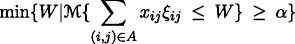which means the shortest length the postman can obtain at confidence level α.

Moreover, we can establish the belief degree maximized-constrained programming model as follows: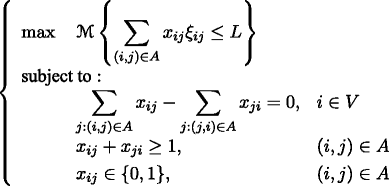((6))

where L is a threshold value of all the route lengths.

### Uncertain optimal assignment problem

In order to model an optimal assignment problem by a graph, some basic concepts of graph theory are introduced, which are from Bondy and Murty .

If the set of vertices of a graph can be partitioned into two subsets X and Y, such that each edge has one vertex in X and one vertex in Y, such a graph is called a bipartite graph. The partition (X,Y) is named a bipartition of the graph. A complete graph is one in which each pair of distinct vertices is joined by an edge. In addition, if a bipartite graph with bipartition (X,Y) in which each vertex of X is joined by exactly one edge to each vertex of Y, such a graph is called a complete bipartite graph.

Assume that n workers are available for n jobs in a company, and each worker is qualified for all of these jobs. Consider an uncertain weighted complete bipartite graph with bipartition (X,Y, ξ), where X={x 1,x 2, ,x n }, Y={y 1,y 2,,y n }, and ξ={ξ ij |x i X,y j Y} is the uncertain weight of the edge. Each ξ ij indicates the uncertain profit made by worker x i on job y j . If the profits of the workers in different jobs are different, how can the decision maker design an assignment plan such that the total profit made by all workers is maximized? This is so-called optimal assignment problem. We also suppose that ξ ij are independent uncertain variables with uncertainty distributions Φ ij , i,j=1,2,,n, respectively.

In order to model the abovementioned uncertain optimal assignment problem, the following decision variables are employed

$$\begin{array}{c} x_{ij}=\left\{\begin{array}{l} 1,\quad\text{if worker $$x_{i}$$ is assigned to job $$y_{j}$$} \\[0.2cm] 0,\quad\text{otherwise.}\end{array}\right. \end{array}$$

As a simple choice, Zhang and Peng  built the following expected value model,

$$\begin{array}{c} \left\{\begin{array}{l} \max\quad E\left[{\sum\limits_{1\leq i,j\leq n}x_{ij}\xi_{ij}}\right] \\ \text{subject to}:\\ \quad\quad\; {\sum\limits_{1\leq j\leq n}x_{ij}=1},\quad i=1,2,\cdots,n\\ \quad\quad\; {\sum\limits_{1\leq i\leq n}x_{ij}=1},\quad j=1,2,\cdots,n\\ \quad\quad\;\: x_{ij}\in\{0,1\},\quad i,j=1,2,\cdots,n \end{array}\right. \end{array}$$
((7))

where the first constraint indicates that every worker is assigned to exactly one job, and the second constraint means that every job is assigned exactly one worker.

Sometimes the decision maker would like to set the specific value as the profit target for pursuit. In the uncertain environment, the target profit may not be obtained in some situations. It is natural that the decision maker would accept the inability to reach the target profit to some extent. However, the target profit must be achieved at a given confidence level. Based on this idea, Zhang and Peng  proposed the α-optimal assignment model pursing the maximal profit at the uncertain measure not less than a predetermined level. The α-optimal assignment model is as follows: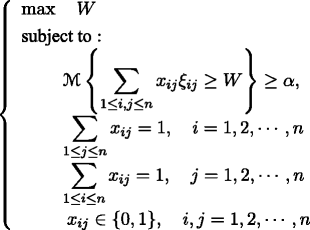((8))

where W is the α-optimal profit defined as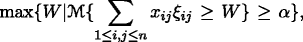the second constraint requires that every worker is assigned to exactly one job, and the third constraint requires that every job is assigned to exactly one worker.

If the decision maker gives the target profit T first, then he/she will want to maximize the belief degree that the uncertain profit is no less than the given target profit. According to this idea, the belief degree maximized-constrained programming model is built as follows: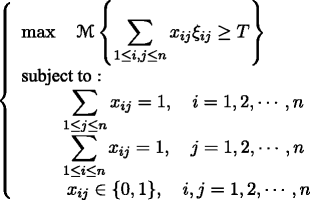((9))

where T is the predetermined target profit.

### Uncertain transportation problem

It is well-known that the transportation problem is a classical network optimization problem that has been researched for many years. As a problem in the uncertain environment, uncertain transportation problems have been studied by Cui and Sheng , Sheng and Yao [61,62] and Zhang and Peng  in recent years. Let us take the fixed charge solid transportation problem as an example.

As a generalization of traditional transportation problem, the solid transportation problem involves how to transport products from m sources to n destinations by l conveyances so that the total transportation cost is minimized. The fixed charge transportation consider two types of costs, including the direct cost and the fixed charge. The direct cost is the cost with respect to per unit transportation amount. When the transportation activity between a source and a destination by a conveyance occurs, the fixed charge will be paid. Denote x ijk as the quantity transported from source i to destination j by conveyance k. The corresponding uncertain cost of unit amount and fixed charge are denoted as ξ ijk and η ijk , respectively. Let $$\tilde {a}_{i}$$ be the amount of products in source i which can be transported to n destinations, $$\tilde {b}_{j}$$ the minimal demand of products in destination j, and $$\tilde {c}_{k}$$ the capacity of conveyance k.

To structure the mathematical program, the following notations are employed:

$$\begin{array}{c} y_{ijk}=\left\{\begin{array}{l} 1,\quad\text{if }\:x_{ijk}>0 \\[0.1cm] 0,\quad\text{otherwise.}\end{array}\right. \end{array}$$

This implies that if x ijk >0, we must add the fixed charge to the total transportation cost.

For the fixed charge solid transportation problem in the uncertain environment, we assume that $$\tilde {a}_{i},\tilde {b}_{j},\tilde {c}_{k},\xi _{\textit {ijk}}$$, and η ijk are independent uncertain variables. Thus, Zhang and Peng  proposed the expected value model as follows:

$$\left\{\begin{array}{l} \text{min} \quad \displaystyle E\left[{\sum_{i=1}^{m}\sum_{j=1}^{n}\sum_{k=1}^{l}}(\xi_{ijk}x_{ijk}+\eta_{ijk}y_{ijk})\right] \\[0.1cm] \text{subject to}:\\ \quad\quad\;\: E\left[{\sum\limits_{j=1}^{n}\sum\limits_{k=1}^{l}}x_{ijk}-\tilde{a}_{i}\right]\leq 0,\quad i=1,2,\cdots,m\\[0.35cm] \quad\quad\;\: E\left[{\sum\limits_{i=1}^{m}\sum\limits_{k=1}^{l}}x_{ijk}-\tilde{b}_{j}\right]\geq 0,\quad j=1,2,\cdots,n\\[0.35cm] \quad\quad\;\: E\left[{\sum\limits_{i=1}^{m}\sum\limits_{j=1}^{n}}x_{ijk}-\tilde{c}_{k}\right]\leq 0,\quad k=1,2,\cdots,l\\[0.35cm] \quad\quad\;\: x_{ijk}\geq 0,\quad y_{ijk}\in\{0,1\} \\[0.1cm] \quad\quad\;\: i=1,2,\cdots,m;\quad j=1,2,\cdots,n;\quad k=1,2,\cdots,l. \end{array}\right.$$
((10))

Under other conditions, the decision maker prefers treating the problem under the belief degree-constraints. This fact provides a motivation for belief degree-constraint programming model founded by Zhang and Peng  as follows: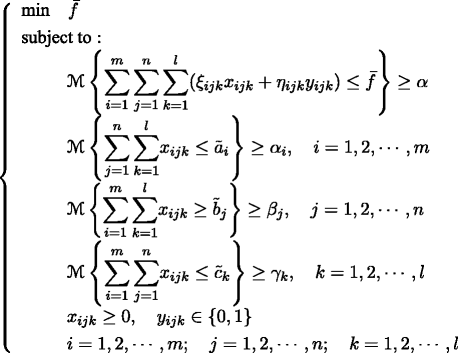((11))

where $$\bar {f}$$ is the α-optimal plan defined as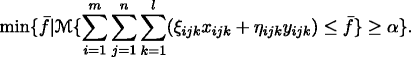In addition, the belief degree maximized-constrained programming model  can be built in the following way,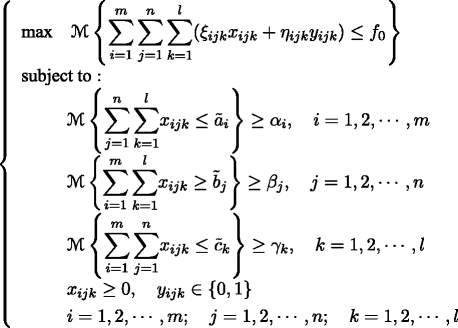((12))

where f 0 is the predetermined value.

## Hybrid intelligent algorithm

In some special cases, we can convert the uncertain programming models into the corresponding crisp equivalents. But this is not the case in most situations due to the computational complexity of the distributions of uncertain objective function or constrained function. Nevertheless, if the uncertain functions are complex, then a suitable hybrid intelligent algorithm may be designed to do this work based on 99-method and genetic algorithm. In the algorithm, 99-method is used as uncertain simulation techniques for calculating the expected value, uncertain measure, and critical value, and genetic algorithm is employed for finding the optimal solution.

### 99-method

The 99-method was proposed by Liu . It is suggested that an uncertain variable ξ i with uncertainty distribution Φ i can be represented by a 99-table (see Table 1). In the first row, 0.01,0.02,,0.99 are the values of uncertainty distribution Φ i of ξ i . In the second row, $${t_{1}^{i}},{t_{2}^{i}},\cdots,t_{99}^{i}$$ are the corresponding values of inverse uncertainty distribution $$\Phi ^{-1}_{i}$$. Let ξ=(ξ 1,ξ 2,,ξ n ), x=(x 1,x 2,,x n ), and f(x; ξ)=f(x;ξ 1,ξ 2,,ξ n ) be a strictly monotone increasing function with respect to ξ 1,ξ 2,,ξ n . Assume that ξ i are independent uncertain variables with uncertainty distributions Φ i , i=1,2,,n, respectively. According to Theorem 2, the inverse uncertainty distribution of f(x; ξ) is $$\Phi _{f}^{-1}(\textbf {x};\alpha)=f(x;\Phi _{1}^{-1}(\alpha),\Phi _{2}^{-1}(\alpha),\cdots,\Phi _{n}^{-1}(\alpha))$$. In the following, we will introduce the methods for calculation expected value E[f(x; ξ)], uncertain measure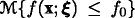, and critical value $$\bar {f}$$ such that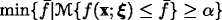.

#### Expected value

It follows from Theorem 2 that the inverse uncertainty distribution of f(x; ξ) is

$$\Phi_{f}^{-1}(\textbf{x};\alpha)=f(\textbf{x};\Phi_{1}^{-1}(\alpha),\Phi_{2}^{-1}(\alpha),\cdots,\Phi_{n}^{-1}(\alpha)),$$

which can be expressed in the computer as Table 2.

By using Theorem 1, we have

$$E[f(\textbf{x};\text{\boldmath{$$\xi$$}})]={\int_{0}^{1}}f(\textbf{x};\Phi_{1}^{-1}(\alpha), \Phi_{2}^{-1}(\alpha),\cdots,\Phi_{n}^{-1}(\alpha))\mathrm{d}\alpha.$$

Thus we design the procedure for computing E[f(x; ξ)] as follows:

Step 1: Set E=0 and j=1.

Step 2: Calculate $$y_{j}=0.01f(\textbf {x};{t_{j}^{1}},{t_{j}^{2}},\cdots,{t_{j}^{n}})$$, and let E:=E+y j .

Step 3: If j<99, let j:=j+1. Turn back to Step 2.

Step 4: Report E as the estimation of E[f(x; ξ)].

#### Uncertain measure

As discussed above, the inverse uncertainty distribution $$\Phi _{f}^{-1}(\textbf {x};\alpha)$$ of f(x; ξ) can be expressed as Table 2. Now, the uncertain measure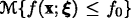can be approximately obtained by the following method

Step 1: Set i=1.

Step 2: Calculate $$y_{i}=f(\textbf {x};{t_{i}^{1}},{t_{i}^{2}},\cdots,{t_{i}^{n}})$$.

Step 3: If y i <f 0, then ii+1, and go back to Step 2.

Step 4: Report α=0.01i as the estimation of the uncertain measure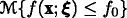.

#### Critical value

Given a predetermined confidence level 0<α<1, we need to find the critical value $$\bar {f}$$ such that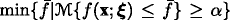. Since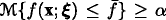can be transformed as $$\Phi _{f}^{-1}(\textbf {x};\alpha)\leq \bar {f}$$. Thus, for any 0<α<1, the critical value $$\bar {f}$$ can be approximately obtained by

$$\frac{\Phi_{f}^{-1}(\textbf{x};0.01\lfloor100\alpha\rfloor)+\Phi_{f}^{-1}(\textbf{x};0.01\lceil100\alpha\rceil)}{2}.$$

### Hybrid intelligent algorithm

As we know, genetic algorithm was first introduced by Holland  and has been developed by many scholars. Many experiments show that genetic algorithm is effective to solve many optimization problems that are difficult to solve by traditional methods. In order to solve the proposed uncertain network models in a numerical method, it is feasible to employ the so-called hybrid intelligent algorithm, which combines 99-method with genetic algorithm. In the algorithm, the uncertain values are calculated by 99-method, and alternately the numerical results are embedded into the genetic algorithm for finding the optimal solution.

Now, the hybrid intelligent algorithm for solving the uncertain network optimization problems is summarized as follows.

Step 1: Initialize p o p s i z e chromosomes at random. Calculate the constraint values and check the feasibility of the chromosomes by 99-method.

Step 2: Update the chromosomes by crossover and mutation operations.

Step 3: Calculate the objective values of the chromosomes by 99-method.

Step 4: Compute the fitness of each chromosome via the objective values, which can be obtained by 99-method.

Step 5: Select the chromosomes by spanning the roulette wheel.

Step 6: Repeat Step 2 to Step 5 for a given number of cycles.

Step 7: Take the best chromosome as the solution of the problems.

## Further research problems

Generally speaking, network optimization problem can be classified into two categories: Network optimization with an uncertain topology structure (i.e., uncertain graph) and network optimization with uncertain weights.

Recently, much attention has been paid to the latter research field. The cited references on uncertain network optimization in this paper almost belong to this area. However, it is no doubt that there are many problems in the uncertain graph needed to be considered, such as tree, regular and vertex degrees, etc. In addition, the network optimization with uncertain weights can be further divided into the following three aspects: 1) Network optimization with uncertain edge weights; 2) Network optimization with uncertain node weights; and 3) Network optimization with uncertain both edge and node weights. As far as we know, the second and the third aspects have been seldom studied up to now.

## Concluding remarks

The uncertain network optimization problem arises from various applications in real life, which is of both theoretical interest in mathematics and applied aspect in practice. Due to the existence of uncertainty almost everywhere and almost every time, uncertain network optimization will be widely applied in such various disciplines. In order to deal with the uncertainty in network optimization, uncertainty theory is employed to model an uncertain network optimization problem.

It is important to keep the following three things in mind: 1) Many network optimization problems appear indeed in an uncertain world; 2) Uncertainty theory provides an efficient tool for dealing with uncertain network optimization problems. 3) There are three types of models for uncertain network optimization with uncertain weights: Expected value model and belief degree-constrained programming model, as well as belief degree maximized-constrained programming model.

In a review manner, this paper presented a comprehensive summary on uncertain network optimization and exhibited the general methods for modeling uncertain network optimization problems. In summary, uncertain network optimization is a new field of interdisciplinary research with opportunities and challenges.

## References

1. Lawler, E: Combinatorial Optimization: Networks and Matroids. Holt, Reinhardt and Winston, New York (2001).

2. Lee, J: A first course in combinatorial optimization. Cambridge University Press, Cambridge (2004).

3. Dreyfus, S: An appraisal of some shortest path algorithms. Oper. Res. 17(3), 395–412 (1969).

4. Graham, RL, Hell, P: On the history of the minimum spanning tree problem. IEEE Ann. Hist. Comput. 7(1), 43–57 (1985).

5. Haley, KB: New methods in mathematical programming–the solid transportation problem. Oper. Res. 10(4), 448–463 (1962).

6. Kuhn, HW: The Hungarian method for the assignment problem. Naval. Res. Logist. Quart. 2(1–2), 83–97 (1955).

7. Mazzoni, G, Pallottino, S, Scutella, MG: The maximum flow problem: a max-preflow approach. Eur. J. Oper. Res. 53(3), 257–278 (1991).

8. Williams, A: A stochastic transportation problem. Oper. Res. 11(5), 759–770 (1963).

9. Donath, WE: Algorithm and average-value bounds for assignment problems. IBM J. Res. Dev. 13(4), 380–386 (1969).

10. Hall, RW: The fastest path through a network with random time-dependent travel times. Transport. Sci. 20(3), 182–188 (1986).

11. Nawathe, SP, Rao, BV: Maximum flow in probabilistic communication networks. Int. J. Circ. Theor. App. 8(2), 167–177 (1980).

12. Mulvey, JM, Vladimirou, H: Stochastic network optimization models for investment planning. Ann. Oper. Res. 20, 187–217 (1989).

13. Neely, MJ: Stochastic network optimization with application to communication and queueing systems. Morgan & Claypool Publishers, San Rafael (2010).

14. Peer, SK, Sharma, DK: Finding the shortest path in stochastic networks. Comput. Math. Appl. 53(5), 729–740 (2007).

15. Ji, Z, Kim, Y, Chen, A: Multi-objective α-reliable path finding in stochastic networks with correlated link costs: a simulation-based multi-objective genetic algorithm approach (SMOGA). Expert Syst. Appl. 38(3), 1515–1528 (2011).

16. Chanas, S, Kolodziejczyk, W: Real-valued flows in a network with fuzzy arc capacities. Fuzzy Sets Syst. 13(2), 139–151 (1984).

17. Hanebeck, UD, Schmidt, GK: Genetic optimization of fuzzy networks. Fuzzy Sets Syst. 79(1), 59–68 (1996).

18. Wu, H: Fuzzy optimization problems based on the embedding theorem and possibility and necessity measures. Math. Comput. Model. 40(3–4), 329–336 (2004).

19. Liu, B: Uncertainty Theory. 4th edn. Springer, Berlin (2015).

20. Liu, B: Why is there a need for uncertainty theory. J. Uncertain Syst. 6(1), 3–10 (2012).

21. Liu, B: Uncertainty Theory. 2nd edn. Springer, Berlin (2007).

22. Liu, B: Uncertain set theory and uncertain inference rule with application to uncertain control. J. Uncertain Syst. 4(2), 83–98 (2010).

23. Liu, B: Uncertain risk analysis and uncertain reliability analysis. J. Uncertain Syst. 4(3), 163–170 (2010).

24. Liu, B: Some research problems in uncertainty theory. J. Uncertain Syst. 3(1), 3–10 (2009).

25. Yao, K: Uncertain calculus with renewal process. Fuzzy Optimization Decis. Mak. 11(3), 258–297 (2012).

26. Liu, B: Fuzzy process, hybrid process and uncertain process. J. Uncertain Syst. 2(1), 3–16 (2008).

27. Chen, X, Liu, B: Existence and uniqueness theorem for uncertain differential equations. Fuzzy Optimization Decis. Mak. 9(1), 69–81 (2010).

28. Yao, K: Extreme values and integral of solution of uncertain differential equation. J. Uncertainty Anal. Appl.1, Article 2 (2013).

29. Liu, B: Toward uncertain finance theory. J. Uncertainty Anal. Appl.1, Article 1 (2013).

30. Peng, J: Risk metrics of loss function for uncertain system. Fuzzy Optimizational Decis. Mak. 12(1), 53–64 (2013).

31. Peng, J, Yao, K: A new option pricing model for stocks in uncertainty markets. Int. J. Oper. Res. 8(2), 18–26 (2011).

32. Gao, X, Gao, Y, Ralescu, DA: On Liu’s inference rule for uncertain systems. Int. J. Uncertainty Fuzziness Knowl.-Based Syst. 18(1), 1–11 (2010).

33. Gao, X, Gao, Y: Connectedness index of uncertain graph. Int. J. Uncertainty Fuzziness Knowledge-Based Syst. 21(1), 127–137 (2013).

34. Zhang, B, Peng, J: Euler index in uncertain graph. Appl. Math. Comput. 218(20), 10279–10288 (2012).

35. Zhang, B, Peng, J: Connectedness strength of two vertices in an uncertain graph. Int. J. Comput. Math. 90(2), 246–257 (2013).

36. Liu, B: Theory and Practice of Uncertain Programming. 2nd edn. Springer, Berlin (2009).

37. Yan, L: Optimal portfolio selection models with uncertain returns. Modern Appl. Sci. 3(8), 76–81 (2009).

38. Rong, L: Two new uncertainty programming models of inventory with uncertain costs. J. Inf. Comput. Sci. 8(2), 280–288 (2011).

39. Gao, Y: Shortest path problem with uncertain arc lengths. Comput. Math. Appl. 62(6), 2591–2600 (2011).

40. Zhang, X, Chen, X: A new uncertain programming model for project scheduling problem. Information. 15(10), 3901–3910 (2012).

41. Ding, S: A new uncertain programming model for grain supply chain design. Information. 16(2), 1069–1076 (2013).

42. Gao, Y: Uncertain models for single facility location problems on networks. Appl. Math. Model. 36(6), 2592–2599 (2012).

43. Zhang, X, Meng, G: Expected-variance-entropy model for uncertain parallel machine scheduling. Information. 16(2), 903–908 (2013).

44. Zhang, X, Wang, Q, Zhou, J: A chance-constrained programming model for inverse spanning tree problem with uncertain edge weights. Int. J. Adv. Comput. Technol. 5(6), 76–83 (2013).

45. Zhang, X, Wang, Q, Zhou, J: Two uncertain programming models for inverse minimum spanning tree problem. Ind. Eng. Manag. Syst. 12(1), 9–15 (2013).

46. Gao, X: Cycle index of uncertain graph. Information. 16(2), 1131–1138 (2013).

47. Zhang, B, Peng, J: Hamilton index and its algorithm of uncertain graph (2014). http://orsc.edu.cn/online/120531.pdf.

48. Zhang, B, Peng, J: Matching index of an uncertain graph: concept and algorithm. Appl. Comput. Math. 12(3), 381–391 (2013).

49. Gao, Y, Yang, L, Li, S, Kar, S: On distribution function of the diameter in uncertain graph, http://orsc.edu.cn/online/131014.pdf (2014).

50. Zhang, B, Peng, J: Uncertain programming model for Chinese postman problem with uncertain weights. Ind. Eng. Manag. Syst. 11(1), 18–25 (2012).

51. Han, S, Peng, Z, Wang, S: The maximum flow problem of uncertain network. Inform. Sci. 265, 167–175 (2014).

52. Ding, S: Uncertain minimum cost flow problem. Soft Comput. 18(11), 2201–2207 (2014).

53. Zhang, B, Peng, J: Uncertain programming model for uncertain optimal assignment problem. Appl. Math. Model. 37(9), 6458–6468 (2013).

54. Zhang, B, Peng, J: Goal programming models for k-cardinality uncertain assignment problem, http://orsc.edu.cn/online/120420.pdf (2014).

55. Zhou, J, He, X, Wang, K: Uncertain quadratic minimum spanning tree problem. J. Commun. 9(5), 385–390 (2014).

56. Zhou, J, Chen, L, Wang, K: Path optimality conditions for minimum spanning tree problem with uncertain edge weights, http://www.orsc.edu.cn/online/131223.pdf (2014).

57. Zhou, J, Yang, F, Wang, K: An inverse shortest path problem on an uncertain graph. J. Networks. 9(9), 2353–2359 (2014).

58. Liu, B: Uncertainty theory: a branch of mathematics for modeling human uncertainty. Springer, Berlin (2010).

59. Newman, MEJ: Networks: An Introduction. Oxford University Press, Oxford (2010).

60. Kolmogorov, AN: Grundbegriffe der Wahrscheinlichkeitsrechnung. Julius Springer, Berlin (1933).

61. Sheng, Y, Yao, K: A transportation model with uncertain costs and demands. Information. 15(8), 3179–3186 (2012).

62. Sheng, Y, Yao, K: Fixed charge transportation problem in uncertain environment. Ind. Eng. Manag. Syst. 11(2), 183–187 (2012).

63. Zhang, B, Peng, J: Uncertain programming models for fixed charge solid transportation problem, http://orsc.edu.cn/online/120828.pdf (2014).

64. Kahneman, D, Tversky, A: Prospect theory: an analysis of decision under risk. Econometrica. 47(2), 263–292 (1979).

65. Bondy, J, Murty, U: Graph theory with applications. Elsevier, New York (1976).

66. Zhang, B, Peng, J: Expected value model for optimal assignment problem with uncertain profits, http://orsc.edu.cn/online/111114.pdf (2014).

67. Cui, Q, Sheng, Y: Uncertain programming model for solid transportation problem. Information. 16(2), 1207–1214 (2013).

68. Holland, J: Adaptation in Natural and Artificial Systems. University of Michigan Press, Ann Arbor (1975).

## Acknowledgements

This work is supported by the Projects of the Humanity and Social Science Foundation of Ministry of Education of China (No. 13YJA630065), the Key Project of Hubei Provincial Natural Science Foundation (No. 2012FFA065), and the Scientific and Technological Innovation Team Project (No. T201110) of Hubei Provincial Department of Education, China.

## Author information

Authors

### Corresponding author

Correspondence to Jin Peng.

### Competing interests

The authors declare that they have no competing interests.

### Authors’ contributions

JP proposed the framework of the study and drafted the manuscript. BZ conceived of the study and participated in its design and coordination. SL participated in the design of the study. All authors read and approved the final manuscript.

## Rights and permissions